# Smith theory of group actions

A collection of techniques and results first obtained by P.A. Smith around 1940 (see [a5], [a6], [a7]) in the area of finite transformation groups. Smith theory is now (2000) best understood via cohomological methods, following an approach introduced by A. Borel (see [a2], [a3]).

The main goal of Smith theory is to study actions of finite-groups on familiar and accessible spaces such as polyhedra or manifolds (cf. also Action of a group on a manifold;-group). However, it can easily be adapted to a very large class of spaces, the so-called finitistic spaces. These are spaces such that every covering has a finite-dimensional refinement (see [a4], p. 133, for details; see also General topology; Covering (of a set)). The most important examples are compact spaces and finite-dimensional spaces. The spaces occurring below are assumed to be of this type.

Letbe such a finitistic space and letbe a finite-group acting on it (hereis a fixed prime number). Let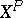be the fixed-point set of the action, that is,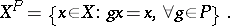The two basic theorems of Smith theory are as follows:

a) Ifhas thehomology of a point (cf. also Homology), then the fixed-point setalso has the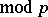homology of a point; in particular, it is non-empty.

b) Ifhas thehomology of a sphere, then the fixed-point set(possibly empty) also has thehomology of a sphere.

Of course, the main examples here are when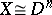, the-dimensional disc, and when, the-dimensional sphere. However, thehomological nature of the results are important, as they can fail at other prime numbers.

Homological methods building on Smith's original approach can be used to verify very general restrictions associated to actions of finite-groups. For example, ifsatisfies the additional hypothesis that its totalcohomology is finite, then there is an inequality arising from an action of a finite-groupon: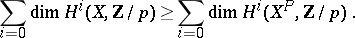Note that this implies that the fixed-point sethas finitely many components and that each of them has finitecohomology. The two previous results can be derived from this inequality.

Another important result which follows from Smith theory is the fact that ifis a finite group acting on a spacewhich is finitistic and acyclic (i.e. has the integral homology of a point), then the orbit space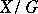is also acyclic.

Smith theory can be considered a precursor to the general cohomological theory of transformation groups (cf. also Transformation group). Given a finite groupacting on a space, one constructs a space, called the Borel construction on, as follows: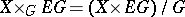, whereis a free, contractible-space. The projection induces a bundle mapping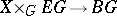, where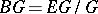is the so-called classifying space of, an Eilenberg–MacLane space of type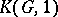. The analysis of this bundle and related constructions is the basic tool in this area. In particular, the main results from Smith theory follow from considering the case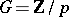; ifis an-dimensional complex with a-action, then the inclusion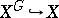induces an isomorphism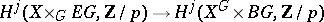provided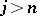. This fact, combined with the spectral sequence incohomology associated to the fibration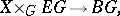are the two main elements used in this reformulation of Smith theory.

See [a1], [a4] and [a8] for excellent references regarding Smith theory and transformation groups.